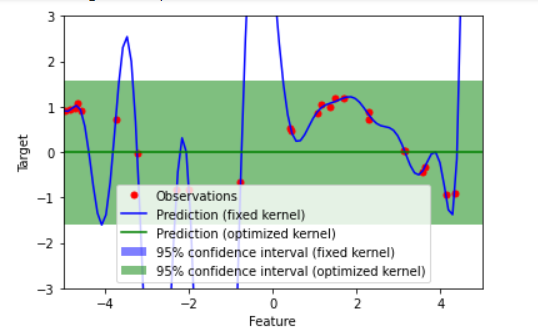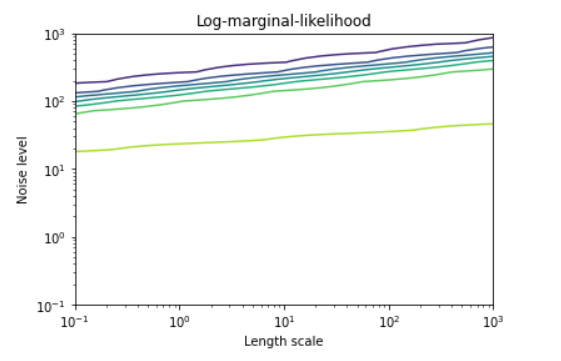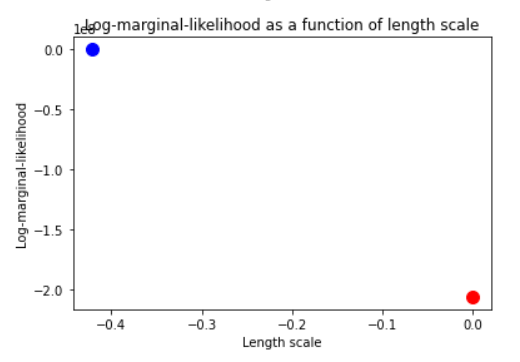GeeksforGeeks App
Open AppBrowser
Continue

# Probabilistic Predictions with Gaussian Process Classification (GPC) in Scikit Learn

Gaussian Process Classification (GPC) is a probabilistic model for classification tasks. It is based on the idea of using a Gaussian process to model the relationship between the input features and the target labels of a classification problem. GPC makes use of Bayesian inference to make predictions, which means that it can output not only the most likely class label for input but also a measure of the uncertainty of the prediction.

### How GPC works?

GPC models the relationship between the input features and the target labels as a Gaussian process, which is a generalization of the Gaussian distribution to a function. Given a set of input features, the GPC model estimates the posterior distribution over the possible target labels. This distribution can then be used to make probabilistic predictions, i.e., to predict not only the most likely class label for a given input but also the uncertainty of the prediction.

One of the main advantages of GPC is that it can output probabilistic predictions, which can be useful in cases where it is important to know not only the most likely class label for a given input, but also the uncertainty of the prediction.

GPC is also a flexible model that can be used with a variety of kernel functions, which allows it to capture a wide range of relationships between the input features and the target labels.

GPC has the ability to automatically tune the hyperparameters of the kernel function through optimization, which can improve the model’s performance. However, GPC can be computationally expensive to train, especially for large datasets, and it is not well-suited for very high-dimensional datasets.

### GPC in Scikit-learn

Installation of Scikit-learn: To install Scikit-learn,  you can use pip, the Python package manager. To install the latest stable version of Scikit-learn, you can run the following command:

`pip install -U scikit-learn`

Importing GPC from Scikit-learn: To use GPC in your Python code, you will need to import the GaussianProcessClassifier class from Scikit-learn’s gaussian_process module. You can do this by adding the following line to the top of your Python file:

`from sklearn.gaussian_process import GaussianProcessClassifier`

Problem is to fit a sine curve to a set of noisy observations using Gaussian Process (GP) regression with fixed and optimized hyperparameters and to visualize the predictions and the log marginal likelihood (LML ) landscape of the optimized GP model. The LML landscape is a contour plot that shows how the LML changes as a function of the kernel hyperparameters. The LML is a measure of the model fit and is used to select the kernel hyperparameters that maximize the LML. The code generates a  dataset of 25 noisy observations of a sine curve and fits a GP model to this data with fixed and optimized hyperparameters. It then makes predictions on a finer grid of points and plots the predictions and the 95% confidence intervals of the predictions. Finally, it plots the LML landscape and the LML as a function of the length scale hyperparameter.

### Import Libraries

Python libraries make it very easy for us to handle the data and perform typical and complex tasks with a single line of code.

• Pandas – This library helps to load the data frame in a 2D array format and has multiple functions to perform analysis tasks in one go.
• Numpy – Numpy arrays are very fast and can perform large computations in a very short time.
• Matplotlib/Seaborn – This library is used to draw visualizations.
• Sklearn – This module contains multiple libraries having pre-implemented functions to perform tasks from data preprocessing to model development and evaluation.

## Python3

 `import` `numpy as np``import` `matplotlib.pyplot as plt``from` `sklearn.gaussian_process ``import` `GaussianProcessRegressor``from` `sklearn.gaussian_process.kernels ``import` `RBF,\``ConstantKernel as C`

Generate the dataset. The dataset is generated using the random number generator (rng) with seed 0. 25 samples are taken from a uniform distribution between -5 and 5 and stored as the feature matrix X. The target variable y is generated as the sin of X and then adding a normally distributed random noise with a mean of 0 and standard deviation of 0.1.

## Python3

 `# Generate data``rng ``=` `np.random.default_rng(``0``)``X ``=` `rng.uniform(``-``5``, ``5``, ``25``)[:, np.newaxis]``y ``=` `np.sin(X).ravel()``y ``+``=` `rng.normal(``0``, ``0.1``, y.size)`

Specify a Gaussian Process Regressor with a fixed kernel (RBF with a fixed length scale of 1.0) and another GP regressor with an RBF kernel with an optimized length scale. Fit the GP models to the observations using the fit method.

## Python3

 `# Specify Gaussian Processes with fixed and optimized hyperparameters``gp_fix ``=` `GaussianProcessRegressor(kernel``=``1.0` `*` `RBF(length_scale``=``1.0``),``                                  ``optimizer``=``None``)``gp_fix.fit(X, y)`` ` `gp_opt ``=` `GaussianProcessRegressor(kernel``=``1.0` `*` `RBF(length_scale``=``1.0``))``gp_opt.fit(X, y)`

Gaussian Processes with fixed and optimized hyperparameters are created and fitted to the X and y data. Predictions are made for X_ with y_fix and y_opt as the mean predictions and sigma_fix and sigma_opt as the standard deviation of the predictions.

## Python3

 `# Make predictions``X_ ``=` `np.linspace(``-``5``, ``5``, ``100``)[:, np.newaxis]``y_fix, sigma_fix ``=` `gp_fix.predict(X_, return_std``=``True``)``y_opt, sigma_opt ``=` `gp_opt.predict(X_, return_std``=``True``)`

Plots the results of the Gaussian process regression with fixed and optimized hyperparameters, including the observed data points, predictions, and 95% confidence intervals. The x-axis represents the feature, while the y-axis represents the target. The plot shows two sets of filled curves, one in blue and one in green, which represent the 95% confidence intervals for the predictions made with the fixed and optimized kernels, respectively. The plot also shows two lines, one in blue and one in green, which represents the mean predictions made with the fixed and optimized kernels, respectively.

## Python3

 `# Plot results``plt.figure()``plt.plot(X, y, ``"r."``, markersize``=``10``, label``=``"Observations"``)``plt.plot(X_, y_fix, ``"b-"``, label``=``"Prediction (fixed kernel)"``)``plt.plot(X_, y_opt, ``"g-"``, label``=``"Prediction (optimized kernel)"``)``plt.fill(``    ``np.concatenate([X_, X_[::``-``1``]]),``    ``np.concatenate([y_fix ``-` `1.9600` `*` `sigma_fix,``                    ``(y_fix ``+` `1.9600` `*` `sigma_fix)[::``-``1``]]),``    ``alpha``=``.``5``,``    ``fc``=``"b"``,``    ``ec``=``"None"``,``    ``label``=``"95% confidence interval (fixed kernel)"``,``)``plt.fill(``    ``np.concatenate([X_, X_[::``-``1``]]),``    ``np.concatenate([y_opt ``-` `1.9600` `*` `sigma_opt,``                    ``(y_opt ``+` `1.9600` `*` `sigma_opt)[::``-``1``]]),``    ``alpha``=``.``5``,``    ``fc``=``"g"``,``    ``ec``=``"None"``,``    ``label``=``"95% confidence interval (optimized kernel)"``,``)``plt.xlabel(``"Feature"``)``plt.ylabel(``"Target"``)``plt.xlim(``-``5``, ``5``)``plt.ylim(``-``3``, ``3``)``plt.legend(loc``=``"best"``)`

Output:95% confidence interval for the optimized kernel models

The above plot shows the observations (red dots) and the predictions made by the GP models with fixed and optimized kernel hyperparameters (blue and green lines, respectively). The blue and green shaded regions show the 95% confidence intervals of the predictions made by the fixed and optimized kernel models, respectively.

Plot a contour plot of log marginal likelihood (LML) with respect to length scale and noise level. The optimized kernel hyperparameters and fixed kernel hyperparameters are indicated with a red dot and a blue circle, respectively, on the plot.

## Python3

 `# Plot LML landscape``plt.figure()``theta0 ``=` `np.logspace(``-``1``, ``3``, ``30``)``theta1 ``=` `np.logspace(``-``1``, ``3``, ``29``)``Theta0, Theta1 ``=` `np.meshgrid(theta0, theta1)``LML ``=` `[``    ``[``        ``gp_opt.log_marginal_likelihood(np.log([Theta0[i, j],``                                               ``Theta1[i, j]]))``        ``for` `i ``in` `range``(Theta0.shape[``0``])``    ``]``    ``for` `j ``in` `range``(Theta0.shape[``1``])``]``LML ``=` `np.array(LML).T``plt.contour(Theta0, Theta1, LML)``plt.scatter(``    ``gp_opt.kernel_.theta[``0``],``    ``gp_opt.kernel_.theta[``1``],``    ``c``=``"r"``,``    ``s``=``50``,``    ``zorder``=``10``,``    ``edgecolors``=``(``0``, ``0``, ``0``),``)``plt.plot(``    ``[gp_fix.kernel_.theta[``0``]],``    ``[gp_fix.kernel_.theta[``1``]],``    ``"bo"``,``    ``ms``=``10``,``)``plt.xscale(``"log"``)``plt.yscale(``"log"``)``plt.xlabel(``"Length scale"``)``plt.ylabel(``"Noise level"``)``plt.title(``"Log-marginal-likelihood"``)`

Output:Contour plot for Log Maximum Likelihood

The above plot is a contour plot of the log marginal likelihood (LML) landscape of the optimized GP model. The red dot shows the optimized kernel hyperparameters, and the blue dot shows the fixed kernel hyperparameters.

Plot the log-marginal-likelihood as a function of the length scale for both the fixed and optimized Gaussian Process models. The log-marginal-likelihood is plotted as a function of the length scale for each model, with the optimized model shown in blue and the fixed model shown in red.

## Python3

 `# Plot LML as a function of length scale``plt.figure()``plt.plot(``    ``gp_opt.kernel_.theta[``0``],``    ``gp_opt.log_marginal_likelihood(gp_opt.kernel_.theta),``    ``"bo"``,``    ``ms``=``10``,``)``plt.plot(``    ``gp_fix.kernel_.theta[``0``],``    ``gp_fix.log_marginal_likelihood(gp_fix.kernel_.theta),``    ``"ro"``,``    ``ms``=``10``,``)``plt.xlabel(``"Length scale"``)``plt.ylabel(``"Log-marginal-likelihood"``)``plt.title("Log``-``marginal``-``likelihood as\``a function of length scale")`` ` `plt.show()`

Output:Length Scale Hyperparameter for model optimization

The above plot shows the LML as a function of the length scale hyperparameter for the optimized (blue dot) and fixed (red dot) kernel models.

My Personal Notes arrow_drop_up